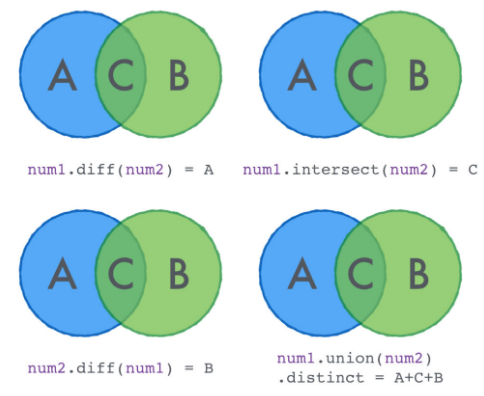# 十个惊人的Scala集合操作函数

categories:资料  author:

本文我将集中介绍几个日常工作必备的 Scala 集合函数，如转换函数和聚合函数。文章最后，我会展示如何结合这些函数以解决具体问题。

## 最大值和最小值

 `int``[] arr = {``11``, ``2``, ``5``, ``1``, ``6``, ``3``, ``9``};` ` ` `int` `to = arr.length - ``1``;` `int` `max = arr[``0``];` ` ` `for` `(``int` `i = ``0``; i < to; i++) {` `    ``if` `(max < arr[i+``1``])` `        ``max = arr[i+``1``];` `}` ` ` `System.out.println(max);`

Scala 推荐了一个很赞的解决方案：

 `val` `numbers ``=` `Seq(``11``, ``2``, ``5``, ``1``, ``6``, ``3``, ``9``) ` `numbers.max ``//11 ` `numbers.min ``//1`

 `case` `class` `Book(title``:` `String, pages``:` `Int)` ` ` `val` `books ``=` `Seq(` `  ``Book(``"Future of Scala developers"``, ``85``),` `  ``Book(``"Parallel algorithms"``, ``240``),` `  ``Book(``"Object Oriented Programming"``, ``130``),` `  ``Book(``"Mobile Development"``, ``495``)` `)` ` ` `//Book(Mobile Development,495)` `books.maxBy(book ``=``> book.pages)` ` ` `//Book(Future of Scala developers,85)` `books.minBy(book ``=``> book.pages)`

## Filter

 `val` `numbers ``=` `Seq(``1``,``2``,``3``,``4``,``5``,``6``,``7``,``8``,``9``,``10``)` `numbers.filter(n ``=``> n ``%` `2` `==` `0``)`

 `val` `books ``=` `Seq(` `  ``Book(``"Future of Scala developers"``, ``85``),` `  ``Book(``"Parallel algorithms"``, ``240``),` `  ``Book(``"Object Oriented Programming"``, ``130``),` `  ``Book(``"Mobile Development"``, ``495``)` `)` ` ` `books.filter(book ``=``> book.pages >``=` `120``)`

## Flatten O_o

 `val` `abcd ``=` `Seq(``'a'``, ``'b'``, ``'c'``, ``'d'``)` `val` `efgj ``=` `Seq(``'e'``, ``'f'``, ``'g'``, ``'h'``)` `val` `ijkl ``=` `Seq(``'i'``, ``'j'``, ``'k'``, ``'l'``)` `val` `mnop ``=` `Seq(``'m'``, ``'n'``, ``'o'``, ``'p'``)` `val` `qrst ``=` `Seq(``'q'``, ``'r'``, ``'s'``, ``'t'``)` `val` `uvwx ``=` `Seq(``'u'``, ``'v'``, ``'w'``, ``'x'``)` `val` `yz   ``=` `Seq(``'y'``, ``'z'``)` ` ` `val` `alphabet ``=` `Seq(abcd, efgj, ijkl, mnop, qrst, uvwx, yz)` ` ` `// List(a, b, c, d, e, f, g, h, i, j, k, l, m, n, o, p, q, r, s, t, u, v, w, x, y, z)` `alphabet.flatten`

## 欧拉图函数（Euler Diagram函数）

 `val` `num``1` `=` `Seq(``1``, ``2``, ``3``, ``4``, ``5``, ``6``)` `val` `num``2` `=` `Seq(``4``, ``5``, ``6``, ``7``, ``8``, ``9``)` ` ` `//List(1, 2, 3)` `num``1``.diff(num``2``)` ` ` `//List(4, 5, 6)` `num``1``.intersect(num``2``)` ` ` `//List(1, 2, 3, 4, 5, 6, 4, 5, 6, 7, 8, 9)` `num``1``.union(num``2``)`

 `//List(1, 2, 3, 4, 5, 6, 7, 8, 9)` `num``1``.union(num``2``).distinct`## map列表元素

map 是 Scala 集合最常用的一个函数。它的功能十分强大：

 `val` `numbers ``=` `Seq(``1``,``2``,``3``,``4``,``5``,``6``)` ` ` `//List(2, 4, 6, 8, 10, 12)` `numbers.map(n ``=``> n * ``2``)` ` ` `val` `chars ``=` `Seq(``'a'``, ``'b'``, ``'c'``, ``'d'``)` ` ` `//List(A, B, C, D)` `chars.map(ch ``=``> ch.toUpper)`

`map` 函数的逻辑是遍历集合中的元素并对每个元素调用函数。你也可以不调用任何函数，保持返回元素本身，但这样 `map` 无法发挥作用，因为你在映射过后得到的是同样的集合。

## flatMap

 `val` `abcd ``=` `Seq(``'a'``, ``'b'``, ``'c'``, ``'d'``)` ` ` `//List(A, a, B, b, C, c, D, d)` `abcd.flatMap(ch ``=``> List(ch.toUpper, ch))`

## 对整个集合进行条件检查

 `val` `numbers ``=` `Seq(``3``, ``7``, ``2``, ``9``, ``6``, ``5``, ``1``, ``4``, ``2``)` ` ` `//ture` `numbers.forall(n ``=``> n < ``10``)` ` ` `//false` `numbers.forall(n ``=``> n > ``5``)`

## 对集合进行分组

 `val` `numbers ``=` `Seq(``3``, ``7``, ``2``, ``9``, ``6``, ``5``, ``1``, ``4``, ``2``)` ` ` `//(List(2, 6, 4, 2), List(3, 7, 9, 5, 1))` `numbers.partition(n ``=``> n ``%` `2` `==` `0``)`

## Fold?

 `val` `numbers ``=` `Seq(``1``, ``2``, ``3``, ``4``, ``5``)` ` ` `//15` `numbers.foldLeft(``0``)((res, n) ``=``> res + n)`

 `val` `words ``=` `Seq(``"apple"``, ``"dog"``, ``"table"``)` ` ` `//13` `words.foldLeft(``0``)((resultLength, word) ``=``> resultLength + word.length)`

## 您最喜欢的函数

 `def` `theLongest(s``:` `String)``:` `String ``=` `{` `  ``s.split (``"[0-9]"``)` `    ``.filter (``_``.exists (ch ``=``> ch.isUpper))` `    ``.filter (``_``.exists (ch ``=``> ch.isLower))` `    ``.maxBy (``_``.length)` `}`

## 总结

Scala具有令人难以置信的强大的集合API，你可以利用它做很多的事情。 此外，相同的事情可以以不同的方式进行，例如： 上面的欧拉函数例子。 Scala的API是很丰富的，我们需要很多时间和练习来学习它。